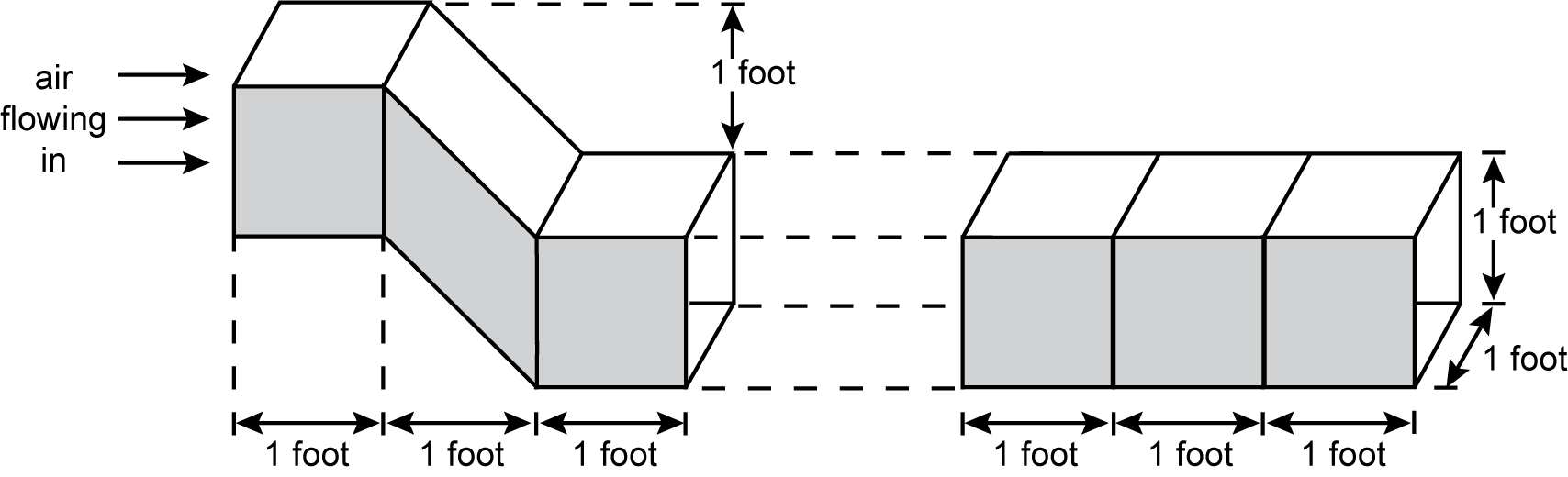﻿ MTEL Test Information Guide

# Test Information Guide

## Field 78: General Curriculum Subtest 2: Mathematics, Science, and Technology/Engineering Sample Multiple-Choice Questions

### Mathematics

##### Objective 0011Apply number theory, structures of numeration systems and operations, and arithmetic properties to the real number system using the Standards for Mathematical Practice.

1. Use the expression below to answer the question that follows.

3545 plus 2456 plus 1505

The value of this sum may be estimated by rounding each of the numbers to a specified place value. One estimate is produced by rounding each of the numbers to the thousands place and another is produced by rounding each of the numbers to the hundreds place. By how much do these estimates differ?

1. 500
2. 600
3. 1400
4. 1500
Correct Response: A.
##### Objective 0014Apply concepts of geometry using the Standards for Mathematical Practice.

2. Use the information below to answer the question that follows.

Two air ducts will be connected so that air flows into the left duct and exits out the right duct. Air enters the left duct by flowing in through a square opening, and the cross section along the lengths of both ducts has this same shape. One side of the ducts, represented by shaded surfaces in the diagram below, will be exposed to warm temperatures.Two segments of air ducts are shown in a horizontal arrangement. Each segment is composed of 3 square prisms that have an open channel running through them that allows air flow to enter from the left side of the left side and exit on the right side of the right segment.

The square prisms all have bases that are 1 foot wide and 1 foot deep. The heights, which are lengths perpendicular to the bases, are also 1 foot long. The 3 square prisms for the left segment are arranged in the following sequence: a right square prism, a square prism that slants down by 1 foot, and a right square prism. This shape carries the airflow 1 foot down and 3 feet right. The right segment is only made of right square prisms, and it carries the airflow 3 feet to the right without any change in height.

An engineer considers the geometry of the ducts to determine how much the air will be warmed by heat entering through the shaded surfaces. Compared to the right duct, the left duct has:

1. the same amount of shaded surface and the same volume.
2. more shaded surface but the same amount of volume.
3. more shaded surface and more volume.
4. less shaded surface and less volume.
Correct Response: A.
##### Objective 0015Apply principles, concepts, and procedures related to measurement and data using the Standards for Mathematical Practice.

3. A ballet dancer prepares to begin a dance by raising their heels until only the tips of their toes are supporting the weight of the dancer's body. A scientist determines that the amount of pressure pressing on the dancer's toes in this position is approximately 220 pounds per square inch. Which of the following values best represents this pressure in pounds per square foot?

1. 1.5 pounds per square foot
2. 18 pounds per square foot
3. 2,640 pounds per square foot
4. 31,680 pounds per square foot
Correct Response: D.

### Science and Technology/Engineering

##### Objective 0016Apply knowledge of Earth and space science principles and the Science and Engineering Practices to interpret and analyze phenomena.

4. Which of the following geological features is primarily formed from chemical weathering processes?

1. barrier islands along the coast
2. valleys and floodplains between mountains
3. sand dunes in the desert
4. underground caves in limestone rocks
Correct Response: D.
##### Objective 0019Apply knowledge of engineering principles and practices to interpret and analyze design problems.

5. A group of students is designing a new type of durable shoe. They give a survey to their classmates that asks about the material and age of the shoes that the students are currently wearing. Which of the following engineering design processes is best represented in this scenario?

1. communicating the test results for a solution
2. modifying and reevaluating solutions
3. providing feedback about a solution
4. researching and generating solutions